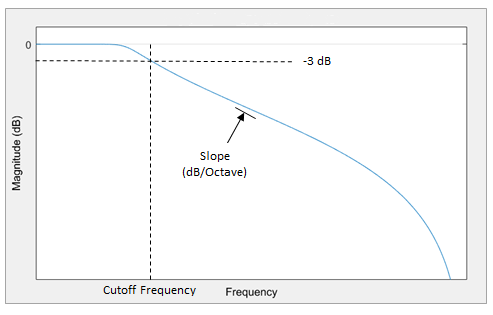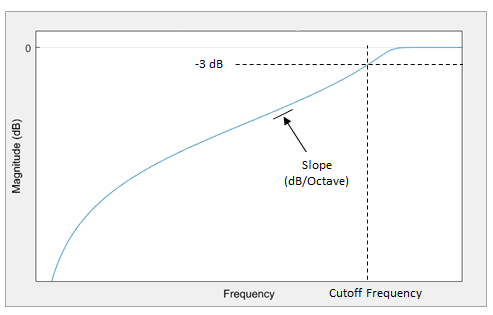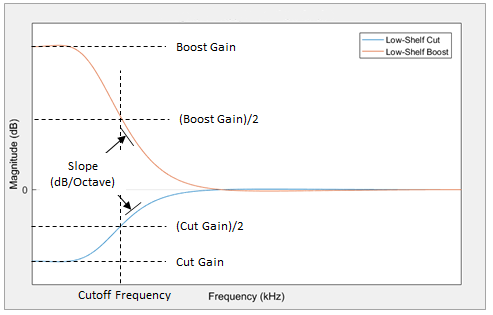## Equalization

Equalization (EQ) is the process of weighting the frequency spectrum of an audio signal.

You can use equalization to:

• Enhance audio recordings

• Analyze spectral content

Types of equalization include:

• Lowpass and highpass filters –– Attenuate high frequency and low frequency content, respectively.

• Low-shelf and high-shelf equalizers –– Boost or cut frequencies equally above or below a desired cutoff point.

• Parametric equalizers –– Selectively boost or cut frequency bands. Also known as peaking filters.

• Graphic equalizers –– Selectively boost or cut octave or fractional octave frequency bands. The bands have standards-based center frequencies. Graphic equalizers are a special case of parametric equalizers.

This tutorial describes how Audio Toolbox™ implements the design functions: `designParamEQ`, `designShelvingEQ`, and `designVarSlopeFilter`. The `multibandParametricEQ` System object™ combines the filter design functions into a multiband parametric equalizer. The `graphicEQ` System object combines the filter design functions and the `octaveFilter` System object for standards-based graphic equalization. For a tutorial focused on using the design functions in MATLAB®, see Parametric Equalizer Design.

### Equalization Design Using Audio Toolbox

Equalizer Type and Design ParametersMagnitude Response Examples

Use `designVarSlopeFilter` to create lowpass and highpass filters.

Design parameters of `designVarSlopeFilter` include:

• Normalized cutoff frequency

• Slope (dB/octave)

LowpassHighpassUse `designShelvingEQ` to create low-shelf and high-shelf equalizers

Design parameters of `designShelvingEQ` include:

• Gain (dB)

• Normalized cutoff frequency

• Slope (dB/octave)

Low-ShelfHigh-ShelfUse `designParamEQ` to create parametric equalizers. You can design single-band parametric equalizers or a cascade of parametric equalizers. Using a cascade of parametric equalizers enables you to tune your frequency response with precision.

Design parameters of `designParamEQ` include:

• Number of equalizer bands

• Gain (dB)

• Normalized bandwidth

• Normalized center frequency

• Filter order

Parametric Equalizer### EQ Filter Design

Audio Toolbox design functions use the bilinear transform method of digital filter design to determine your equalizer coefficients. In the bilinear transform method, you:

1. Choose an analog prototype.

2. Specify filter design parameters.

3. Perform the bilinear transformation.

#### Analog Low-Shelf PrototypeAudio Toolbox uses the high-order parametric equalizer design presented in . In this design method, the analog prototype is taken to be a low-shelf Butterworth filter:

`${H}_{\text{a}}\left(s\right)={\left[\frac{g\beta +s}{\beta +s}\right]}^{r}\prod _{i=1}^{L}\left[\frac{{g}^{2}{\beta }^{2}+2g{s}_{i}\beta s+{s}^{2}}{{\beta }^{2}+2{s}_{i}\beta s+{s}^{2}}\right]$`

$\text{ }\text{ }\text{ }\text{ }\text{ }•\text{ }L$ = Number of analog SOS sections

$\text{ }\text{ }\text{ }\text{ }\text{ }•\text{ }N$ = Analog filter order

$\text{ }\text{ }\text{ }\text{ }\text{ }•\text{ }r=\left\{\begin{array}{c}0\text{\hspace{0.17em}}\text{ }\text{N}\text{\hspace{0.17em}}\text{even}\\ 1\text{ }\text{\hspace{0.17em}}\text{N}\text{\hspace{0.17em}}\text{odd}\text{\hspace{0.17em}}\text{\hspace{0.17em}}\end{array}$

$\text{ }\text{ }\text{ }\text{ }\text{ }•\text{ }g={G}^{1/N}$

$\text{ }\text{ }\text{ }\text{ }\text{ }•\text{ }\beta ={\Omega }_{\text{B}}×{\left(\sqrt{\frac{{G}^{2}-{G}_{\text{B}}^{2}}{{G}_{\text{B}}^{2}-1}}\right)}^{-1}{N}}=\mathrm{tan}\left(\pi \frac{\Delta \omega }{2}\right)×{\left(\sqrt{\frac{{G}^{2}-{G}_{\text{B}}^{\text{2}}}{{G}_{\text{B}}^{2}-1}}\right)}^{-1}{N}}$, where Δω is the desired digital bandwidth

$\text{ }\text{ }\text{ }\text{ }\text{ }•\text{ }{s}_{i}=\mathrm{sin}\left(\frac{\left(2i-1\right)\pi }{2N}\right),\text{ }i=1,2,...,L$

For parametric equalizers, the analog prototype is reduced by setting the bandwidth gain to the square root of the peak gain (GB = sqrt(G)).

After the design parameters are specified, the analog prototype is transformed directly to the desired digital equalizer by a bandpass bilinear transformation:

`$s=\frac{1-2\mathrm{cos}\left({\omega }_{0}\right){z}^{-1}+{z}^{-2}}{1-{z}^{-2}}$`

ω0 is the desired digital center frequency.

This transformation doubles the filter order. Every first-order analog section becomes a second-order digital section. Every second-order analog section becomes a fourth-order digital section. Audio Toolbox always calculates fourth-order digital sections, which means that returning second-order sections requires the computation of roots, and is less efficient.

#### Digital Coefficients

The digital transfer function is implemented as a cascade of second-order and fourth-order sections.

`$H\left(z\right)={\left[\frac{{b}_{00}+{b}_{01}{z}^{-1}+{b}_{02}{z}^{-2}}{1+{a}_{01}{z}^{-1}+{a}_{02}{z}^{-2}}\right]}^{r}\prod _{i=1}^{L}\left[\frac{{b}_{i0}+{b}_{i1}{z}^{-1}+{b}_{i2}{z}^{-2}+{b}_{i3}{z}^{-3}+{b}_{i4}{z}^{-4}}{1+{a}_{i1}{z}^{-1}+{a}_{i2}{z}^{-2}+{a}_{i3}{z}^{-3}+{a}_{i4}{z}^{-4}}\right]$`

The coefficients are given by performing the bandpass bilinear transformation on the analog prototype design.

Second-Order Section CoefficientsFourth-Order Section Coefficients

`$\begin{array}{l}{D}_{0}=\beta +1\\ {b}_{00}=\left(1+g\beta \right)/{D}_{0}\\ {b}_{01}=-2\mathrm{cos}\left({\omega }_{0}\right)/{D}_{0}\\ {b}_{02}=\left(1-g\beta \right)/{D}_{0}\\ {a}_{01}=-2\mathrm{cos}\left({\omega }_{0}\right)/{D}_{0}\\ {a}_{02}=\left(1-\beta \right)/{D}_{0}\end{array}$`

`$\begin{array}{l}{D}_{i}={\beta }^{2}+2{s}_{i}\beta +1\\ {b}_{i0}=\left({g}^{2}{\beta }^{2}+2g{s}_{i}\beta +1\right)/{D}_{i}\\ {b}_{i1}=-4{c}_{0}\left(1+g{s}_{i}\beta \right)/{D}_{i}\\ {b}_{i2}=2\left(1+2{\mathrm{cos}}^{2}\left({\omega }_{0}\right)-{g}^{2}{\beta }^{2}\right)/{D}_{i}\\ {b}_{i3}=-4{c}_{0}\left(1-g{s}_{i}\beta \right)/{D}_{i}\\ {b}_{i4}=\left({g}^{2}{\beta }^{2}-2g{s}_{i}\beta +1\right)/{D}_{i}\\ {a}_{i1}=-4{c}_{0}\left(1+{s}_{i}\beta \right)/{D}_{i}\\ {a}_{i2}=2\left(1+2{\mathrm{cos}}^{2}\left({\omega }_{0}\right)-{\beta }^{2}\right)/{D}_{i}\\ {a}_{i3}=-4\mathrm{cos}\left({\omega }_{0}\right)\left(1-{s}_{i}\beta \right)/{D}_{i}\\ {a}_{i4}=\left({\beta }^{2}-2{s}_{i}\beta +1\right)/{D}_{i}\end{array}$`

Biquadratic Case.  In the biquadratic case, when N = 1, the coefficients reduce to:

`$\begin{array}{l}{D}_{0}=\frac{{\Omega }_{\text{B}}}{\sqrt{G}}+1\\ {b}_{00}=\left(1+{\Omega }_{\text{B}}\sqrt{G}\right)/{D}_{0},\text{ }{b}_{01}=-2\mathrm{cos}\left({\omega }_{0}\right)/{D}_{0},\text{ }{b}_{02}=\left(1-{\Omega }_{\text{B}}\sqrt{G}\right)/{D}_{0}\\ {a}_{01}=-2\mathrm{cos}\left({\omega }_{0}\right)/{D}_{0},\text{ }{a}_{02}=\left(1-\frac{{\Omega }_{\text{B}}}{\sqrt{G}}\right)/{D}_{0}\end{array}$`

Denormalizing the a00 coefficient, and making substitutions of A =sqrt(G), ${\Omega }_{\text{B}}\cong \alpha$ yields the familiar peaking EQ coefficients described in .

Orfanidis notes the approximate equivalence of ΩB and $\alpha$ in .

By using trigonometric identities,

`${\Omega }_{B}=\mathrm{tan}\left(\frac{\Delta \omega }{2}\right)=\mathrm{sin}\left({\omega }_{0}\right)\mathrm{sinh}\left(\frac{\mathrm{ln}2}{2}B\right)\text{\hspace{0.17em}},$`

where B plays the role of an equivalent octave bandwidth.

Bristow-Johnson obtained an approximate solution for B in :

`$B=\frac{{\omega }_{0}}{\mathrm{sin}{\omega }_{0}}×BW$`

Substituting the approximation for B into the ΩB equation yields the definition of $\alpha$ in :

`$\alpha =\mathrm{sin}\left({\omega }_{0}\right)\mathrm{sinh}\left(\frac{\mathrm{ln}2}{2}×\frac{{\omega }_{0}}{\mathrm{sin}{\omega }_{0}}×BW\right)$`

### Lowpass and Highpass Filter Design

#### Analog Low-Shelf Prototype

To design lowpass and highpass filters, Audio Toolbox uses a special case of the filter design for parametric equalizers. In this design, the peak gain, G, is set to 0, and GB2 is set to 0.5 (–3 dB cutoff). The cutoff frequency of the lowpass filter corresponds to 1 – ΩB. The cutoff frequency of the highpass filter corresponds to ΩB.#### Digital Coefficients

The table summarizes the results of the bandpass bilinear transformation. The digital center frequency, ω0, is set to π for lowpass filters and 0 for highpass filters.

Second Order Section CoefficientsFourth Order Section Coefficients

`$\begin{array}{l}{D}_{0}=1+\mathrm{tan}\left(\pi \frac{\Delta \omega }{2}\right)\\ {b}_{00}=1/{D}_{0}\\ {b}_{01}=-2\mathrm{cos}\left({\omega }_{0}\right)/{D}_{0}\\ {b}_{02}=1/{D}_{0}\\ {a}_{01}=-2\mathrm{cos}\left({\omega }_{0}\right)/{D}_{0}\\ {a}_{02}=\left(1-\mathrm{tan}\left(\pi \frac{\Delta \omega }{2}\right)\right)/{D}_{0}\end{array}$`

`$\begin{array}{l}{D}_{i}={\mathrm{tan}}^{2}\left(\pi \frac{\Delta \omega }{2}\right)+2{s}_{i}\mathrm{tan}\left(\pi \frac{\Delta \omega }{2}\right)+1\\ {b}_{i0}=1/{D}_{i}\\ {b}_{i1}=-4\mathrm{cos}\left({\omega }_{0}\right)/{D}_{i}\\ {b}_{i2}=2\left(1+2{\mathrm{cos}}^{2}\left({\omega }_{0}\right)\right)/{D}_{i}\\ {b}_{i3}=-4\mathrm{cos}{\left({\omega }_{0}\right)}_{0}/{D}_{i}\\ {b}_{i4}=1/{D}_{i}\\ {a}_{i1}=-4\mathrm{cos}\left({\omega }_{0}\right)\left(1+{s}_{i}\mathrm{tan}\left(\pi \frac{\Delta \omega }{2}\right)\right)/{D}_{i}\\ {a}_{i2}=2\left(1+2{\mathrm{cos}}^{2}\left({\omega }_{0}\right)-{\mathrm{tan}}^{2}\left(\pi \frac{\Delta \omega }{2}\right)\right)/{D}_{i}\\ {a}_{i3}=-4\mathrm{cos}\left({\omega }_{0}\right)\left(1-{s}_{i}\mathrm{tan}\left(\pi \frac{\Delta \omega }{2}\right)\right)/{D}_{i}\\ {a}_{i4}=\left({\mathrm{tan}}^{2}\left(\pi \frac{\Delta \omega }{2}\right)-2{s}_{i}\mathrm{tan}\left(\pi \frac{\Delta \omega }{2}\right)+1\right)/{D}_{i}\end{array}$`

### Shelving Filter Design

#### Analog Prototype

Audio Toolbox implements the shelving filter design presented in . In this design, the high-shelf and low-shelf analog prototypes are presented separately:

`${H}_{\text{L}}\left(s\right)=A\left(\frac{A{s}^{2}+\left(\sqrt{A}}{Q}\right)s+1}{{s}^{2}+\left(\sqrt{A}}{Q}\right)s+A}\right)\text{ }\text{ }\text{ }\text{ }\text{ }{H}_{\text{H}}\left(s\right)=A\left(\frac{{s}^{2}+\left(\sqrt{A}}{Q}\right)s+A}{A{s}^{2}+\left(\sqrt{A}}{Q}\right)s+1}\right)$`

For compactness, the analog filters are presented with variables A and Q. You can convert A and Q to available Audio Toolbox design parameters:

`$\begin{array}{l}A={10}^{G/40}\\ \frac{1}{Q}=\sqrt{\left(A+1}{A}\right)\left(1}{slope}-1\right)+2}\end{array}$`

After you specify the design parameters, the analog prototype is transformed to the desired digital shelving filter by a bilinear transformation with prewarping:

`$s=\left(\frac{z-1}{z+1}\right)×\left(\frac{1}{\mathrm{tan}\left(\frac{{\omega }_{0}}{2}\right)}\right)$`

#### Digital Coefficients

The table summarizes the results of the bilinear transformation with prewarping.

Low-ShelfHigh-ShelfIntermediate Variables

`$\begin{array}{l}{b}_{0}=A\left(\left(A+1\right)-\left(A-1\right)\mathrm{cos}\left({\omega }_{0}\right)+2\alpha \sqrt{A}\right)\\ {b}_{1}=2A\left(\left(A-1\right)-\left(A+1\right)\mathrm{cos}\left({\omega }_{0}\right)\right)\\ {b}_{2}=A\left(\left(A+1\right)-\left(A-1\right)\mathrm{cos}\left({\omega }_{0}\right)-2\alpha \sqrt{A}\right)\\ {a}_{0}=\left(A+1\right)+\left(A-1\right)\mathrm{cos}\left({\omega }_{0}\right)+2\alpha \sqrt{A}\\ {a}_{1}=-2\left(\left(A-1\right)+\left(A+1\right)\mathrm{cos}\left({\omega }_{0}\right)\right)\\ {a}_{2}=\left(A+1\right)+\left(A-1\right)\mathrm{cos}\left({\omega }_{0}\right)-2\alpha \sqrt{A}\end{array}$`

`$\begin{array}{l}{b}_{0}=A\left(\left(A+1\right)+\left(A-1\right)\mathrm{cos}\left({\omega }_{0}\right)+2\alpha \sqrt{A}\right)\\ {b}_{1}=-2A\left(\left(A-1\right)+\left(A+1\right)\mathrm{cos}\left({\omega }_{0}\right)\right)\\ {b}_{2}=A\left(\left(A+1\right)+\left(A-1\right)\mathrm{cos}\left({\omega }_{0}\right)-2\alpha \sqrt{A}\right)\\ {a}_{0}=\left(A+1\right)-\left(A-1\right)\mathrm{cos}\left({\omega }_{0}\right)+2\alpha \sqrt{A}\\ {a}_{1}=2\left(\left(A-1\right)+\left(A+1\right)\mathrm{cos}\left({\omega }_{0}\right)\right)\\ {a}_{2}=\left(A+1\right)-\left(A-1\right)\mathrm{cos}\left({\omega }_{0}\right)-2\alpha \sqrt{A}\end{array}$`

`$\begin{array}{l}\alpha =\frac{\mathrm{sin}\left({\omega }_{0}\right)}{2}\sqrt{\left(A+\frac{1}{A}\right)\left(\frac{1}{slope}-1\right)+2A}\\ {\omega }_{0}=2\pi \frac{Cutoff\text{\hspace{0.17em}}Frequency}{Fs}\end{array}$`

 Orfanidis, Sophocles J. "High-Order Digital Parametric Equalizer Design." Journal of the Audio Engineering Society. Vol. 53, November 2005, pp. 1026–1046.

 Bristow-Johnson, Robert. "Cookbook Formulae for Audio EQ Biquad Filter Coefficients." Accessed March 02, 2016. http://www.musicdsp.org/files/Audio-EQ-Cookbook.txt.

 Orfanidis, Sophocles J. Introduction to Signal Processing. Englewood Cliffs, NJ: Prentice Hall, 2010.

 Bristow-Johnson, Robert. "The Equivalence of Various Methods of Computing Biquad Coefficients for Audio Parametric Equalizers." Presented at the 97th Convention of the AES, San Francisco, November 1994, AES Preprint 3906.

## SupportGet trial now